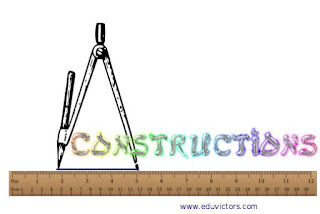## Constructions

Based NCERT Class 9 Mathematics Chapter

Q1: With the help of ruler and compass, which of the following angles can be constructed?

(a) 35°
(b) 40°
(c) 37.5°
(d) 15°

Answer:  37.5° and 15° angles can be constructed using a ruler and a compass.

Q2(NCERT Exemplar): With the help of a ruler and a compass it is not possible to construct an angle of :

(A) 37.5°
(B) 40°
(C) 22.5°
(D) 67.5°

Q3 (NCERT Exemplar): Write True or False in each of the following. Give reasons for your answer:

1. An angle of 52.5° can be constructed.

2. An angle of 42.5° can be constructed.

3. A triangle ABC can be constructed in which AB = 5 cm, ∠A = 45° and BC + AC = 5 cm.

4. A triangle ABC can be constructed in which BC = 6 cm, ∠C = 30° and AC – AB = 4 cm.

5. A triangle ABC can be constructed in which ∠ B = 105°, ∠C = 90° and AB + BC + AC = 10 cm.

6. A triangle ABC can be constructed in which ∠ B = 60°, ∠C = 45° and AB + BC + AC = 12 cm

1: True. Construct 210° (180° + 30°), bisect it two times, 210° ÷ 4 = 52.5°

2: False. Since 85° ÷ 2 = 42.5° and 85° angle cannot be constructed using compass.

3: False. In a △ the sum of any two sides must be greater than the third side. ∵ AB > BC +AC, such a △ cannot be constructed.

4: True. As AC – AB < BC, i.e., AC < AB + BC.

5: False. Using angle sum property of △ i.e. 180°. As ∠B + ∠C = 105° + 90° = 195° >180°.

6. True. Using angle sum property of △ i.e. 180°. As ∠B + ∠C = 60° + 45° = 105° < 180°.

Q4 (Fill in the blanks): A triangle can be constructed when its three _______ are given. (sides/angles)

Q5: What type of triangle can be constructed if one side is known.

Q6(MCQ): With ruler and compass which of the following angles cannot be constructed?

(a) 60°
(b) 80°
(c) 90°
(d) 105°

Q7(MCQ): With a ruler and compass which of the following angles can be constructed?

(a) 110°
(b) 100°
(c) 90°
(d) 80°